CBSE Class 9 Lines and Angles MCQ New

## CBSE Class 9 Lines and Angles MCQ By Clarify Knowledge

CBSE Class 9 Lines and Angles MCQ New Pattern 2022

## CBSE Class 9 Lines and Angles MCQ Table

Question 1.
In ΔABC, ∠A = 50° and the external bisectors of ∠B and ∠C meet at O as shown in figure. The measure of ∠BOC is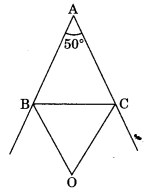(a) 40°
(b) 65°
(c) 115°

Question 2.
In figure the value of x is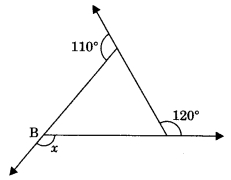(a) 120°
(b) 130°
(c) 110°

Question 3.
An exterior angle of a triangle is 80° and the interior opposite angles are in the ratio 1 : 3, measure of interior opposite angles are
(a) 30°, 90°
(b) 40°, 120°
(c) 20°, 60°

Question 4.
In ΔABC, the bisectors of ∠ABC and ∠BCA intersect each other at O. The measure of ∠BOC is
(a) 90° + ∠A
(b) 90° + ∠A2
(c) 180 – ∠A

Question 5.
In figure if ∠A + ∠B + ∠C + ∠D + ∠E + ∠F = k right angles, then find value of k.(а) 2
(b) 3
(c) 4

Question 6.
In the given figure, the measure of ∠ABC is(a) 80°
(b) 20°
(c) 100°

Question 7.
The angle of a triangle are in the ratio 5 : 3 : 7, the triangle is
(а) an acute-angled triangle
(b) an obtuse angled triangle
(c) an right angled triangle

Question 8.
In figure l1 || l2, the value of x is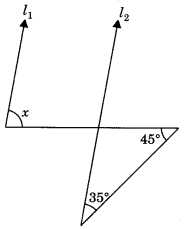(a) 80°
(b) 100°
(c) 110°

Question 9.
If one angle of triangle is equal to the sum of the other two, then the triangle is
(a) an isosceles triangle
(b) an obtuse-angled triangle
(c) an equilateral triangle

Question 10.
One of the angles of a triangle is 75°. If the difference of other two is 35°, then the largest angle of other two angles has a measure
(a) 80°
(b) 75°
(c) 70°

Question 11.
An exterior angle of a triangle is 105° and its two interior opposite angles are equal. Each of these equal angles is
(a) 37 1°2
(b) 52 1°2
(c) 72 1°2

Question 12.
In Figure measure of ∠ABC is(a) 60°
(b) 70°
(c) 80°

Question 13.
In Figure AB || CD, the value of x is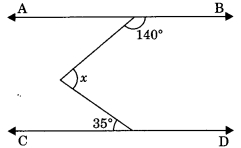(a) 35°
(b) 40°
(c) 60°

Question 14.
In the figure, PS ⊥ l, RQ ⊥ l, the degree measure of y is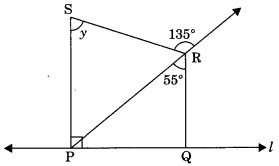(a) 55°
(b) 90°
(c) 80°

Question 15.
Each angle of an equilateral triangle is
(a) 50°
(b) 90°
(c) 54°

Question 16.
Given lines l1 || l2 and l2 || l3 in figure. The value of x is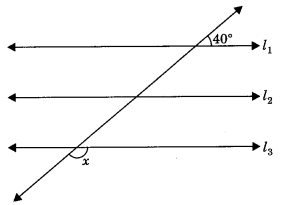(a) 40°
(b) 140°
(c) 50°

Question 17.
In figure, find the value of y.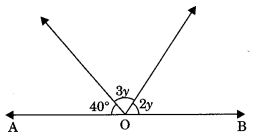(a) 28°
(b) 40°
(c) 140°